Next: Applying the Model Up: Implementation and Interpretation Previous: Implementation and Interpretation

## Conditional Constraints vs. Joint Constraints

We now briefly discuss the difference in constraints on a conditional model versus a joint model. First and foremost, the conditional model (in theory) provides no information about the density in the covariate variables (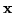). Thus, it does not allocate any resources in modeling thedomain unless it indirectly helps model the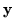domain. Thus, the M Gaussians (i.e. the model's finite resources) do not cluster around the density inunnecessarily.

In addition, note that there are no constraints on the gates to force them to integrate to 1. The mixing proportions are not necessarily normalized and the individual gate models are unnormalized Gaussians. Thus, the gates form an unnormalized marginal density called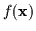which need not integrate to 1. In joint models, on the other hand, the marginal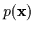must integrate to 1.

Finally, we note that the covariance matrix in the gates is independent of the covariance matrix and regressor matrix in the expert. In a full joint Gaussian, these 3 matrices combine into one large matrix and this matrix as a whole must be symmetric and positive semi-definite. However, here, the gate covariance need not be positive semi-definite. The expert covariance is symmetric positive semi-definite only on its own and the regressor matrix is arbitrary. Thus, the constraints on the total parameters are fewer than in the joint case and each gate-expert combination can model a larger space than a conditioned Gaussian. Thus, training up a conditional model directly will yield solutions that lie outside the space of the conditioned joint models. This is depicted in Figure 7.11. Note how the additional constraints on the joint density limit the realizable conditional models. This limit is not present if the conditional models can be varied in their final parametric form.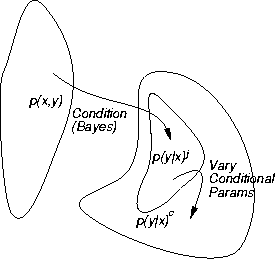Next: Applying the Model Up: Implementation and Interpretation Previous: Implementation and Interpretation
Tony Jebara
1999-09-15